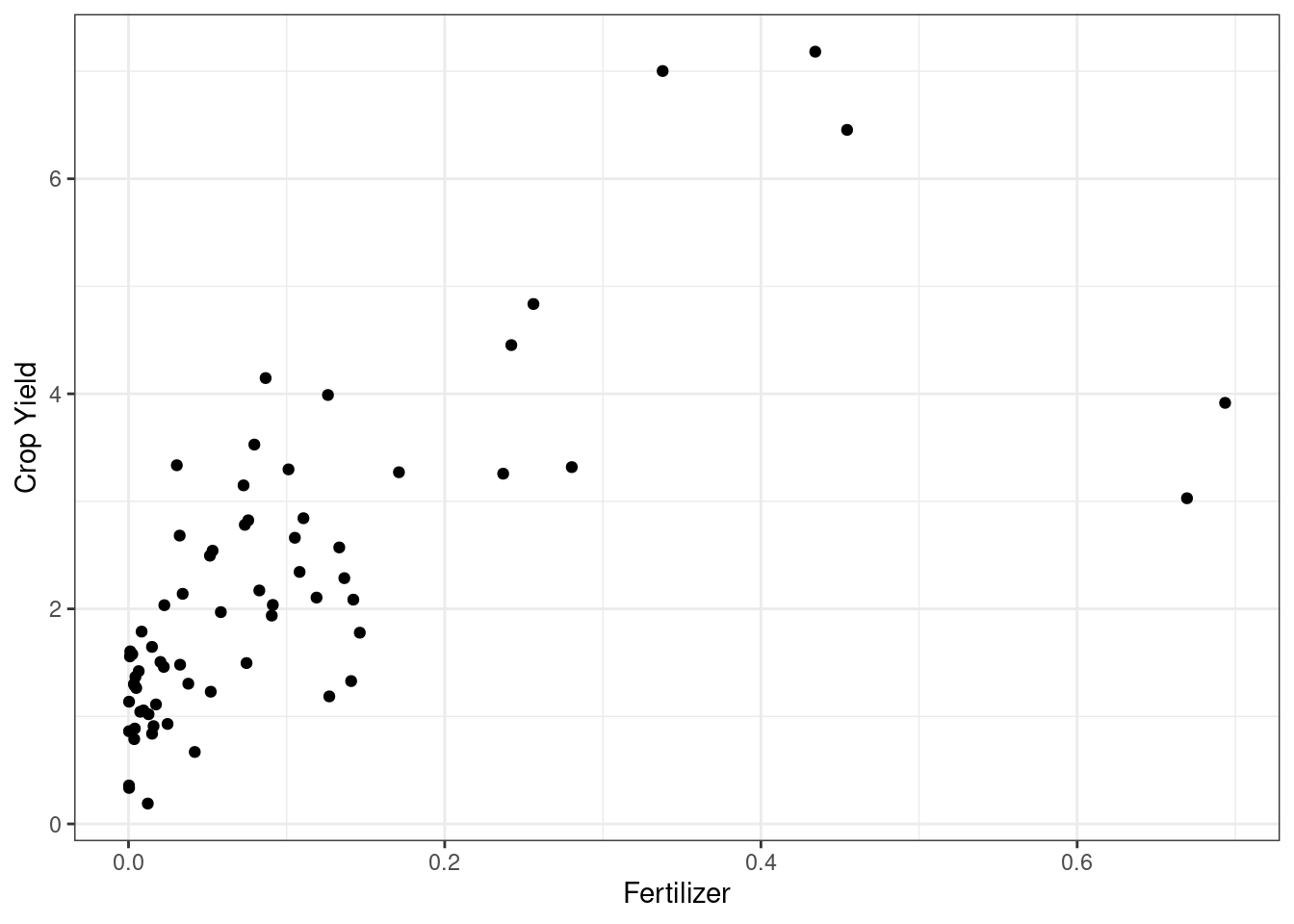## Ch. 3, Coding Question 2

load("fertilizer_2000.RData")
# load packages
library(ggplot2)

# make scatter plot
ggplot(data=fertilizer_2000,
mapping=aes(x=avfert, y=avyield)) +
geom_point() +
ylab("Crop Yield") +
xlab("Fertilizer") +
theme_bw()It seems like countries with higher fertilizer usage tend to have higher crop yields.

## Ch. 4, Extra Question 1

Consistency is a large sample property for an estimator. It says that, if we have a large sample, then our estimator should be close to the population quantity that we are trying to estimate.

An unbiased estimator is one that, if we could repeatedly draw samples of size $$n$$ ($$n$$ could be large or small here) and re-estimate the parameter of interest, then on average (across samples in this repeated sampling thought experiment), our estimate would be equal to the population parameter that we are trying to estimate.

## Ch. 4, Extra Question 2

No, an unbiased estimator does not necessarily have to be consistent. Consider estimating $$\mathbb{E}[Y]$$ by $$Y_1$$ (i.e., using the value of $$Y$$ for the first observation in the data). This estimate of $$\mathbb{E}[Y]$$ is unbiased (since $$\mathbb{E}[Y_1] = \mathbb{E}[Y])$$, but it is not consistent (because it does not even depend on the sample size at all).

## Ch. 4, Extra Question 3

No, consistent estimators can be biased. Consider estimating $$\mathbb{E}[Y]$$ by $$\bar{Y} + \frac{c}{n}$$ where $$c$$ is a constant. This is consistent because $$\bar{Y} \rightarrow \mathbb{E}[Y]$$ as $$n \rightarrow \infty$$ (by the law of large numbers) and $$\frac{c}{n} \rightarrow 0$$ as $$n \rightarrow \infty$$ (because it is a constant divided by a number that is going to infinity). However, notice that

\begin{aligned} \mathbb{E}\left[\bar{Y} + \frac{c}{n} \right] &= \mathbb{E}[Y] + \frac{c}{n} \\ & \neq \mathbb{E}[Y] \end{aligned} so, for any fixed sample size $$n$$, $$\bar{Y} + \frac{c}{n}$$ is biased for $$\mathbb{E}[Y]$$.

## Ch. 4, Extra Question 4

1. Since $$n$$ grows faster than $$\sqrt{n}$$, $$n \left(\frac{1}{n}\sum_{i=1}^n (Y_i - \mathbb{E}[Y])\right)$$ diverges (i.e., the absolute value goes to infinity as $$n \rightarrow \infty$$)

2. Since $$n^{1/3}$$ grows slower than $$\sqrt{n}$$, $$n^{1/3} \left(\frac{1}{n}\sum_{i=1}^n (Y_i - \mathbb{E}[Y])\right)$$ converges to 0 as $$n \rightarrow \infty$$